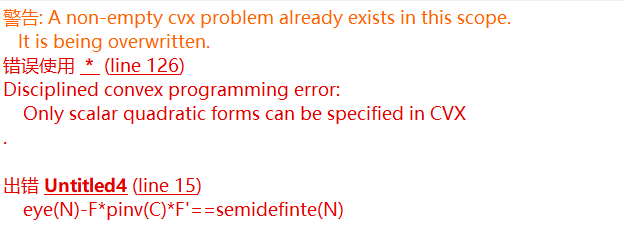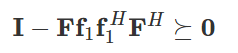# How to express Generalized Schuur complement using CVX?``````clc,clear;
close all;
N = 10;
Nt = 4;

f = rand(Nt,1)+1i*rand(Nt,1);
C = f*f';
cvx_begin
variable F(N,Nt) complex
minimize (norm(F,'fro'))
subject to
real((eye(Nt)-C*pinv(C))*F')==0
eye(N)-F*pinv(C)*F'==semidefinte(N)
cvx_end``````

if you have something of the form of (2) or (3), you enter (1) as a semidefnite constraint, using standard CVX syntax. The whole point of Schur complement is that you do not directly enter semiedefinite constraints in (2) or (3), which are (nonconvex) Bilinear Matrix Inequalities( BMIs). You instead enter semidefinite constraint for (1), which is a linear (convex) SDP…

I believe generalized just refers to possible use of pseudo inverse rather than inverse in the case of a psd matrix which is singular (not strictly positive definite).

i have the constraint，where F is the optimization variable, i want to convert this constraint to LMI using Generalized Schuu complement.

This is just a standard Schur complement, with `A = eye(N), B = F*f, C = 1`. which matches (3). Hence enter (1) in CVX.

`[eye(N) F*f;(F*f)' 1] == hermitian_semidefinite(N+1)`

ok,thank you very much.

Whoops. Make that hermitian_semidefinite(N+1), which I just corrected (N+1, not N).

Or use sdp mode, in which case can you can just use `...>= 0`. I don’t know why with ==semidefinite, CVX makes you put in the dimension. It ought to be able to figure out the dimension itself, as it does in sdp mode.

ok,thank you very much.